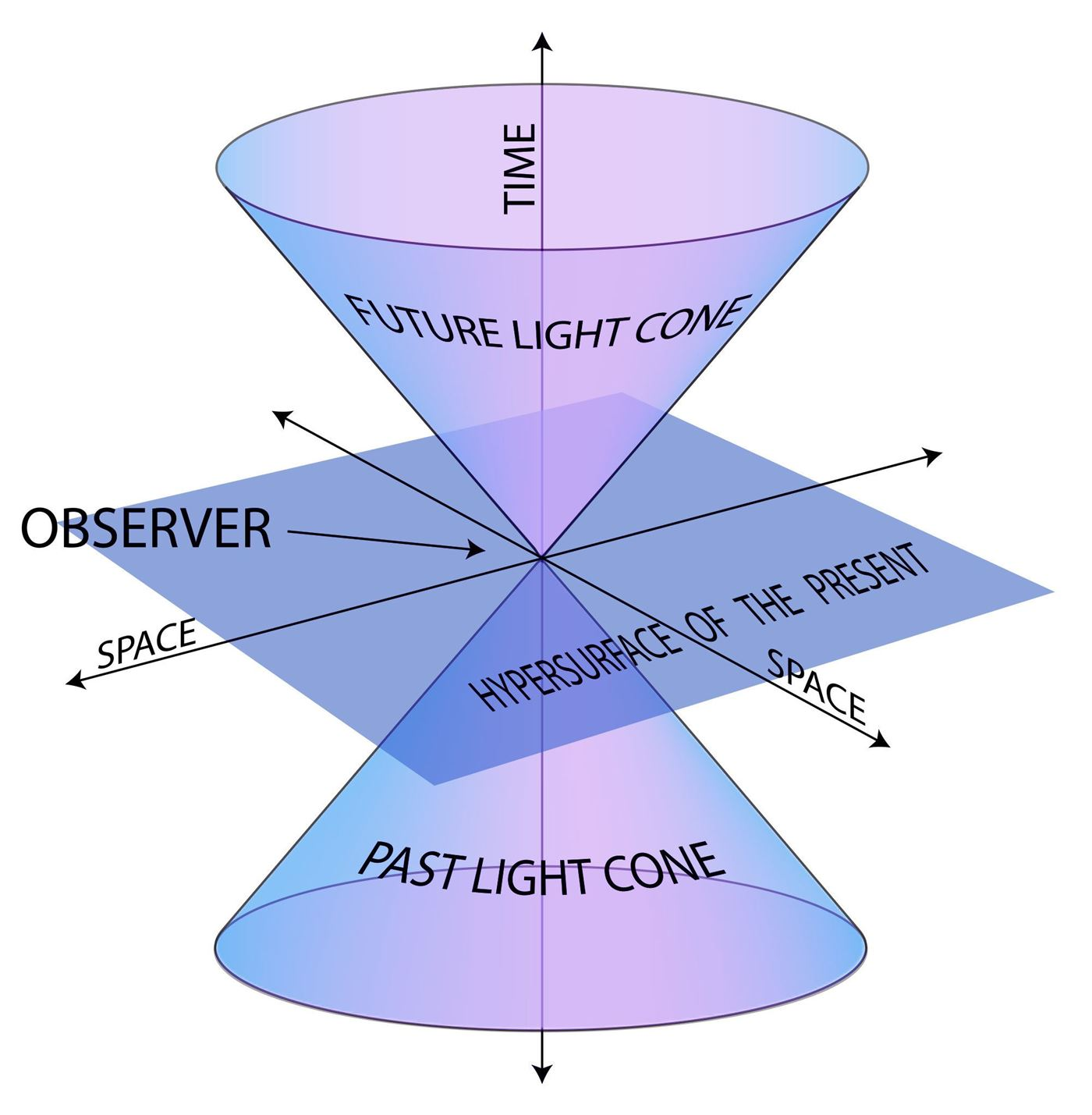# Special Theory of Relativity

The special theory of relativity or special relativity is a physical theory which states the relationship between space and time. This is often termed as STR theory. Special theory of relativity is based on two postulates –

1. Laws of Physics are invariant
2. Irrespective of the light source, the speed of light in a vacuum is the same in any other space.

Albert Einstein originally proposed this theory in the year 1905 “On the Electrodynamics of Moving Bodies”. Special relativity implies consequences of mass-energy equivalence, relativity of simultaneity, length contraction, and a universal speed limit. The conventional notion of absolute universal time is replaced by the notion of a time that is dependent on the reference frame and spatial position.In relative theory, reference frames play a vital role. It is used to measure a time of events by using a clock. An event is nothing but an occurrence that refers to a location in space corresponding to the reference frame. For instance, the explosion of a fire flower can be considered as an event.

## Postulates of Special Relativity

 The Principle of Relativity “ The states of changed physical systems are not affected, whether these changes of state be referred to the one or the other of two systems in uniform translatory motion relative to each other.” The Principle of Invariant Light Speed “Light is always propagated in empty space with a definite velocity c which is independent of the state of motion of the emitting body.”

The origin of Special Relativity not only depends upon these two postulates, but there are other factors such as the clock from their previous history and isotropy.

## Relativity and Unifying Electromagnetism

Wave propagation was discovered during the theoretical investigation in classical electromagnetism. Classical electromagnetism is a branch of theoretical physics that mainly deals with the synergy between electric charges and current by using the classical Newtonian model. The theory provides an explanation of electromagnetic phenomena whenever the field strengths and relevant length scales are large enough that quantum mechanical effects are negligible. The broad study of moving charges forms the Liénard–Wiechert potential, leading to further development towards special theory of relativity.

## Frequently Asked Questions – FAQs

### Who developed the special theory of relativity?

Special theory of relativity was developed by Albert Einstein.

### State Einstein’s equation which shows energy and mass are interchangeable.?

Einstein’s equation is E = mc^2.

### Speed of light is represented using which alphabet?

Speed of light is represented using the letter ‘c’.

### State third law of Newton’s motion?

Third law of Newton’s motion states that “for every action, there is an equal and opposite reaction”.

### Can light travel in vacuum?

Yes, light is an electromagnetic wave which can travel in vacuum.

Test your knowledge on Special Theory Of Relativity